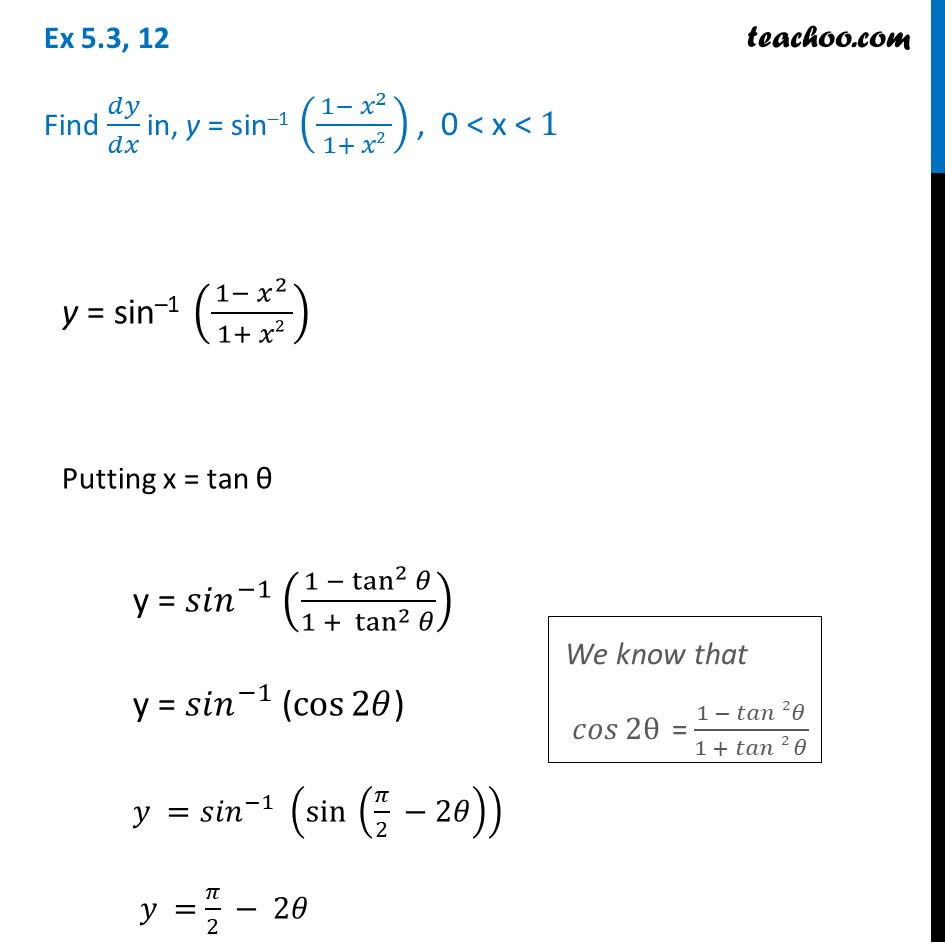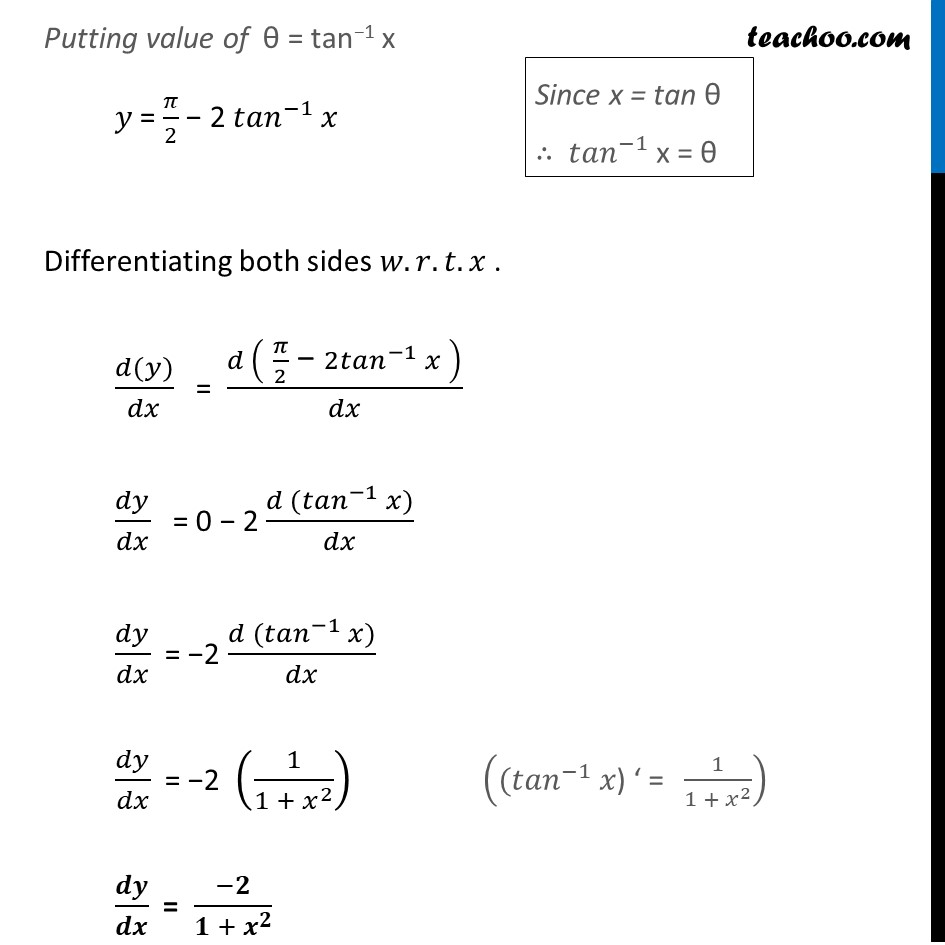Ex 5.3

Chapter 5 Class 12 Continuity and Differentiability
Serial order wiseLearn in your speed, with individual attention - Teachoo Maths 1-on-1 Class

### Transcript

Ex 5.3, 12 Find 𝑑𝑦/𝑑𝑥 in, y = sin–1 ((1− 𝑥^2)/( 1+ 𝑥2 )) , 0 < x < 1 y = sin–1 ((1− 𝑥^2)/( 1+ 𝑥2 )) Putting x = tan θ y = 〖𝑠𝑖𝑛〗^(−1) (〖1 − tan^2〗⁡𝜃/〖1 + tan^2〗⁡𝜃 ) y = 〖𝑠𝑖𝑛〗^(−1) (cos⁡2𝜃) 𝑦 =〖𝑠𝑖𝑛〗^(−1) (〖sin 〗⁡(𝜋/2 −2𝜃) ) 𝑦 = 𝜋/2 − 2𝜃 "We know that" " " █(𝑐𝑜𝑠⁡2θ " =" (1 − 𝑡𝑎𝑛⁡2 𝜃)/(1 + 𝑡𝑎𝑛⁡2 𝜃)) Putting value of θ = tan−1 x 𝑦 = 𝜋/2 − 2 〖𝑡𝑎𝑛〗^(−1) 𝑥 Differentiating both sides 𝑤.𝑟.𝑡.𝑥 . (𝑑(𝑦))/𝑑𝑥 = (𝑑 (" " 𝜋/2 " − " 2〖𝑡𝑎𝑛〗^(−1) 𝑥" " ))/𝑑𝑥 𝑑𝑦/𝑑𝑥 = 0 − 2 (𝑑〖 (𝑡𝑎𝑛〗^(−1) 𝑥))/𝑑𝑥 𝑑𝑦/𝑑𝑥 = −2 (𝑑〖 (𝑡𝑎𝑛〗^(−1) 𝑥))/𝑑𝑥 𝑑𝑦/𝑑𝑥 = −2 (1/(1 + 𝑥^2 )) 𝒅𝒚/𝒅𝒙 = (−𝟐)/(𝟏 + 𝒙^𝟐 ) Since x = tan θ ∴ 〖𝑡𝑎𝑛〗^(−1) x = θ ((〖𝑡𝑎𝑛〗^(−1) 𝑥") ‘ = " 1/(1 + 𝑥^2 ))